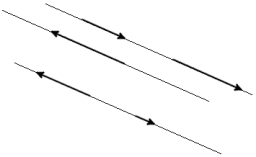# Proportion 20443

On line AB, 15 cm long, point C lies 4 cm from point A. In what proportion does this point divide the line AB?

r =  4:11

### Step-by-step explanation:Did you find an error or inaccuracy? Feel free to write us. Thank you!

Tips for related online calculators
Need help calculating sum, simplifying, or multiplying fractions? Try our fraction calculator.
Check out our ratio calculator.
Do you want to convert length units?